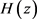Grover量子搜索算法中的最速降线问题研究Study on Brachistochrone Problem for Grover’s Quantum Search Algorithm

• 全文下载: PDF(493KB)    PP.455-460   DOI: 10.12677/APP.2018.811058
• 下载量: 166  浏览量: 265

Grover量子搜索算法是近二十年最著名的量子算法，其已经被证明无法被任何经典算法所超越，展示出极强的量子加速特性。Grover量子搜索算法可以被两种等价的途径所实现，即线路模型Grover算法和绝热Grover算法。本文从绝热Grover算法的角度出发，运用变分法中广为人知的最速降线问题来探讨Grover量子搜索算法，得到了其量子加速特性的一个必要原因，这使得按照该算法的实质来设计其他量子算法成为可能。

Grover’s quantum search algorithm is the most famous quantum algorithm in the last twenty years, which exhibits a strong quantum speedup and has been proved not to be able to be outperformed by any classical algorithm. Grover’s quantum search algorithm can be implemented by two equivalent ways, which are circuit model Grover’s algorithm and adiabatic Grover’s algorithm, and the latter is the start point of the paper. We here apply the well-known brachistochrone problem in calculus of variations to investigate and obtain a necessary condition of Grover’s quantum search algorithm, which makes possible to design new efficient quantum algorithms.

1. 引言

1982年，Feynman提出了量子计算机的想法，其目的是克服经典计算机在模拟量子力学过程中经典算法本质上存在的困难  。1994年，Shor提出了量子计算机的第一个量子算法  ，用于求极大整数的因子分解，其效率明显优于目前的最先进的经典因子分解算法，但无法证明不存在经典算法比Shor量子因子分解算法更高效。1997年，Grover量子搜索算法横空出世  ，打破了人们关于经典算法可以替代量子算法的疑惑  。Grover量子搜索算法可由量子线路模型和绝热量子计算两种等价的方法实现。简单地讲，量子线路模型通过各种通用量子酉门的时序组合来编码并求解得到最终结果；而绝热量子计算则通过制备初始哈密顿及其基态，并将结果编码进查询哈密顿的基态，在量子绝热定理的保证下来得到结果。本文从绝热量子计算角度来探讨Grover量子搜索算法，得到了其量子加速特性的一个必要原因，这一结果使得按照该算法的实质来设计其他量子算法成为可能。

2. 绝热Grover算法

${H}_{i}=1-|\varphi 〉〈\varphi |$

${H}_{q}=1-|q〉〈q|$

$H\left(z\right)=\left(1-z\right){H}_{i}+z{H}_{q}$

$H\left(z\right)=\frac{1}{2}-\frac{1}{2}\left(\begin{array}{cc}-1+2\left(1-z\right)\left(1-\frac{1}{N}\right)& 2\left(1-z\right)\frac{1}{\sqrt{N}}\sqrt{1-\frac{1}{N}}\\ 2\left(1-z\right)\frac{1}{\sqrt{N}}\sqrt{1-\frac{1}{N}}& 1-2\left(1-z\right)\left(1-\frac{1}{N}\right)\end{array}\right)$

$\begin{array}{l}\Delta \left(z\right)=\sqrt{{\left(1-2z\right)}^{2}+\frac{4}{N}z\left(1-z\right)}\\ \mathrm{cos}\theta \left(z\right)=\frac{1}{\Delta \left(z\right)}\left[1-2\left(1-z\right)\left(1-\frac{1}{N}\right)\right]\\ \mathrm{sin}\theta \left(z\right)=\frac{2}{\Delta \left(z\right)}\left(1-z\right)\frac{1}{\sqrt{N}}\sqrt{1-\frac{1}{N}}\end{array}$

$H\left(z\right)$ 有如下紧凑形式

$H\left(z\right)=\frac{1}{2}+\frac{\Delta \left(z\right)}{2}\left(\begin{array}{cc}\mathrm{cos}\theta \left(z\right)& \mathrm{sin}\theta \left(z\right)\\ \mathrm{sin}\theta \left(z\right)& -\mathrm{cos}\theta \left(z\right)\end{array}\right)$

$\begin{array}{l}{E}_{-}=\frac{1}{2}\left(1-\Delta \left(z\right)\right),\text{\hspace{0.17em}}\text{\hspace{0.17em}}\text{\hspace{0.17em}}|{E}_{-}〉=\mathrm{sin}\frac{\theta \left(z\right)}{2}|{q}^{\perp }〉+\mathrm{cos}\frac{\theta \left(z\right)}{2}|q〉\\ {E}_{+}=\frac{1}{2}\left(1+\Delta \left(z\right)\right),\text{\hspace{0.17em}}\text{\hspace{0.17em}}\text{\hspace{0.17em}}|{E}_{+}〉=\mathrm{cos}\frac{\theta \left(z\right)}{2}|{q}^{\perp }〉-\mathrm{sin}\frac{\theta \left(z\right)}{2}|q〉\end{array}$

$\mathrm{cos}\theta \approx -1,\mathrm{sin}\theta \approx 0$ 意味着 $\theta \approx \text{π}$ ；依据量子绝热定理， $|{E}_{-}〉$ 应始终是绝热演化过程的基态，且当 $z=1$ 时绝热量子系统 $H\left(1\right)={H}_{q}$ 并查询到目标态 $|q〉$ ，相应的系统参数为 $\Delta =1,\mathrm{cos}\theta =1,\mathrm{sin}\theta =0$ ，意味着 $\theta =0\mathrm{mod}2\text{π}$ 。由于绝热演化的基态 $|{E}_{-}〉$ 是绝热量子计算唯一关心的态，那么我们将它稍作调整，令 $\theta \left(z\right)=\alpha \left(z\right)+\text{π}$ ，则变为

$H\left(z\right)=\frac{1}{2}-\frac{\Delta \left(z\right)}{2}\left(\begin{array}{cc}\mathrm{cos}\alpha \left(z\right)& \mathrm{sin}\alpha \left(z\right)\\ \mathrm{sin}\alpha \left(z\right)& -\mathrm{cos}\alpha \left(z\right)\right)\end{array}\right)$

$|{E}_{-}〉=\mathrm{cos}\frac{\theta \left(z\right)}{2}|{q}^{\perp }〉-\mathrm{sin}\frac{\theta \left(z\right)}{2}|q〉$ (1)

3. 绝热Grover算法与最速降线问题

$\text{d}{s}^{2}=〈\frac{\text{D}\psi }{\text{d}\tau }|\frac{\text{D}\psi }{\text{d}\tau }〉\text{d}{\tau }^{2}$

$J\left(|\psi \left(\cdot \right)〉\right)=\underset{C}{\int }\text{d}s=\underset{{\tau }_{1}}{\overset{{\tau }_{2}}{\int }}\text{d}\tau ‖\frac{\text{D}\psi }{\text{d}\tau }‖=\underset{{\tau }_{1}}{\overset{{\tau }_{2}}{\int }}\text{d}\tau \sqrt{〈\frac{\text{D}\psi }{\text{d}\tau }|\frac{\text{D}\psi }{\text{d}\tau }〉}$

$\begin{array}{l}\delta J\left(|\psi \left(\cdot \right)〉\right)\\ =\delta \underset{{\tau }_{1}}{\overset{{\tau }_{2}}{\int }}\text{d}\tau \sqrt{〈\frac{\text{D}\psi }{\text{d}\tau }|\frac{\text{D}\psi }{\text{d}\tau }〉}\\ =-\underset{{\tau }_{1}}{\overset{{\tau }_{2}}{\int }}\text{d}\tau \frac{1}{2\sqrt{〈\frac{\text{D}\psi }{\text{d}\tau }|\frac{\text{D}\psi }{\text{d}\tau }〉}}\left[〈\delta \psi |\frac{{\text{D}}^{2}\psi }{\text{d}{\tau }^{2}}〉+〈\frac{{\text{D}}^{2}\psi }{\text{d}{\tau }^{2}}|\delta \psi 〉\right]\end{array}$

$\frac{{\text{D}}^{2}}{\text{d}{\tau }^{2}}|\psi \left(\tau \right)〉+{\omega }^{2}|\psi \left(\tau \right)〉=0$ (2)

${\omega }^{2}=〈\frac{\text{d}\psi \left(\tau \right)}{\text{d}\tau }|\frac{\text{d}\psi \left(\tau \right)}{\text{d}\tau }〉$ ，事实上

$\begin{array}{c}\frac{\text{d}{\omega }^{2}}{\text{d}\tau }=〈\frac{{\text{d}}^{2}\psi \left(\tau \right)}{\text{d}{\tau }^{2}}|\frac{\text{d}\psi \left(\tau \right)}{\text{d}\tau }〉+〈\frac{\text{d}\psi \left(\tau \right)}{\text{d}\tau }|\frac{{\text{d}}^{2}\psi \left(\tau \right)}{\text{d}{\tau }^{2}}〉\\ =〈\frac{{\text{D}}^{2}\psi \left(\tau \right)}{\text{d}{\tau }^{2}}|\frac{\text{d}\psi \left(\tau \right)}{\text{d}\tau }〉+〈\frac{\text{d}\psi \left(\tau \right)}{\text{d}\tau }|\frac{{\text{D}}^{2}\psi \left(\tau \right)}{\text{d}{\tau }^{2}}〉\end{array}$

$\begin{array}{c}={\omega }^{2}〈\psi \left(\tau \right)|\frac{\text{d}\psi \left(\tau \right)}{\text{d}\tau }〉+{\omega }^{2}〈\frac{\text{d}\psi \left(\tau \right)}{\text{d}\tau }|\psi \left(\tau \right)〉\\ =0\end{array}$

$\frac{{{D}^{\prime }}^{2}}{\text{d}{\tau }^{2}}|{\psi }^{\prime }\left(\tau \right)〉+{\omega }^{2}|{\psi }^{\prime }\left(\tau \right)〉=0$

$\frac{{\text{d}}^{2}}{\text{d}{\tau }^{2}}|\psi \left(\tau \right)〉+{\omega }^{2}|\psi \left(\tau \right)〉=0$ (3)

${\tau }_{1}=0,{\tau }_{2}=\frac{3\text{π}}{2\omega }$ ，则对如下常微分方程的边值问题，

$\left\{\begin{array}{l}\frac{{\text{d}}^{2}}{\text{d}{\tau }^{2}}|\psi \left(\tau \right)〉+{\omega }^{2}|\psi \left(\tau \right)〉=0\\ |\psi \left({\tau }_{1}\right)〉=|{\psi }_{1}〉,|\psi \left({\tau }_{2}\right)〉=|{\psi }_{2}〉\end{array}$

$|{\psi }^{o}\left(\tau \right)〉=\mathrm{cos}\omega \tau |{\psi }_{1}〉-\mathrm{sin}\omega \tau |{\psi }_{2}〉$ (4)

4. 结论及展望

  Feynman, R.P. (1982) Simulating Physics with Computers. International Journal of Theoretical Physics, 21, 467. https://doi.org/10.1007/BF02650179  Shor, P. (1994) Algorithms for Quantum Computation: Discrete Loga-rithms and Factoring. Proceedings of the 35th Annual Symposium on Foundations of Computer Science, Santa Fe, 20-22 November 1994, 124. https://doi.org/10.1109/SFCS.1994.365700  Grover, L.K. (1997) Quantum Mechanics Helps in Searching for a Needle in a Haystack. Physical Review Letters, 79, 325. https://doi.org/10.1103/PhysRevLett.79.325  Zalka, C. (1999) Grover’s Quantum Searching Algorithm Is Optimal. Physical Review A, 60, 2746. https://doi.org/10.1103/PhysRevA.60.2746  Roland, J. and Cerf, N.J. (2002) Quantum Search by Local Adi-abatic Evolution. Physical Review A, 65, Article ID: 042308. https://doi.org/10.1103/PhysRevA.65.042308  Cafaro, C. and Mancini, S. (2012) On Grover’s Search Algorithm from a Quantum Information Geometry Viewpoint. Physica A, 391, 1610-1625. https://doi.org/10.1016/j.physa.2011.09.018  Biham, E., et.al. (1999) Grover’s Quantum Search Algorithm for an Arbitrary Initial Amplitude Distribution. Physical Review A, 60, 2742. https://doi.org/10.1103/PhysRevA.60.2742  del Campo, A., et.al. (2013) Quantum Speed Limits in Open System Dynamics. Physical Review Letters, 110, Article ID: 050403. https://doi.org/10.1103/PhysRevLett.110.050403